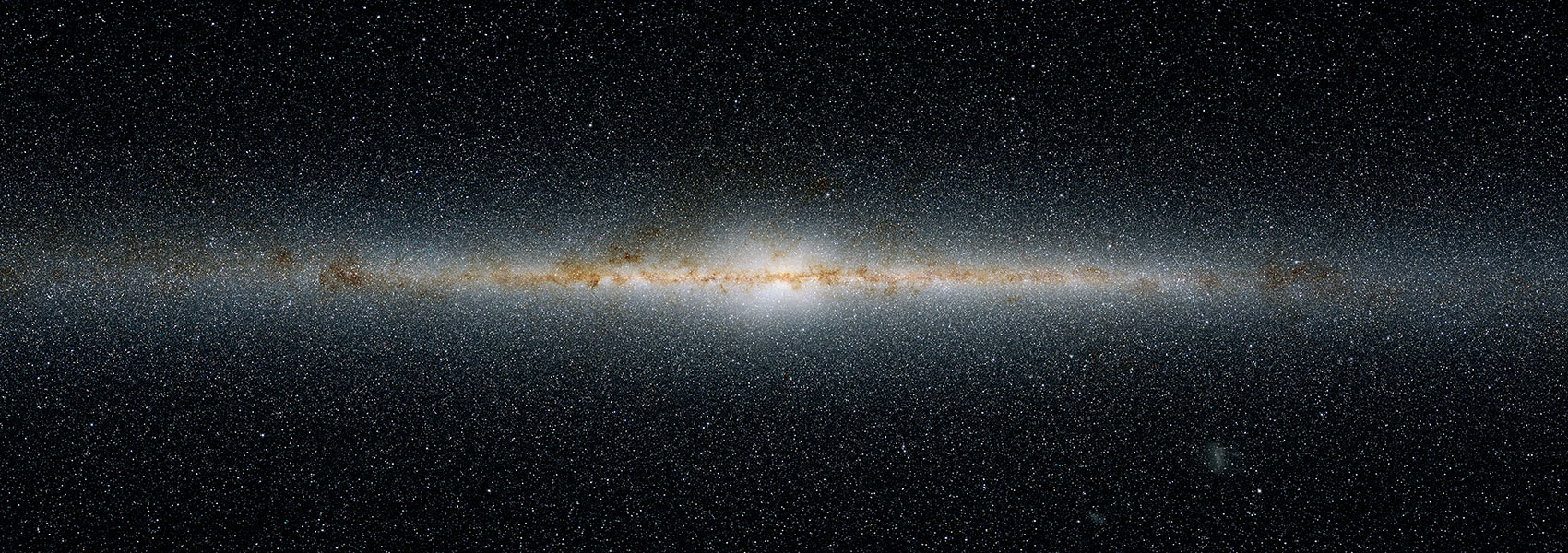# The ALPINE-ALMA [C II] survey: Investigation of 10 galaxies at z 4.5 with [O II] and [C II] line emission - ISM properties and [O II]-SFR relation

March 2022 • 2022MNRAS.511.1303V

Authors • Vanderhoof, Brittany N. • Faisst, A. L. • Shen, L. • Lemaux, B. C. • Béthermin, M. • Capak, P. L. • Cassata, P. • Le Fèvre, O. • Schaerer, D. • Silverman, J. • Yan, L. • Boquien, M. • Gal, R. • Kartaltepe, J. • Lubin, L. M. • Dessauges-Zavadsky, M. • Fudamoto, Y. • Ginolfi, M. • Hathi, N. P. • Jones, G. C. • Koekemoer, A. M. • Narayanan, D. • Romano, M. • Talia, M. • Vergani, D. • Zamorani, G.

Abstract • We present 10 main-sequence ALPINE galaxies (log (M/M) = 9.2-11.1 and ${\rm SFR}=23-190\, {\rm M_{\odot }\, yr^{-1}}$) at z ~ 4.5 with optical [O II] measurements from Keck/MOSFIRE spectroscopy and Subaru/MOIRCS narrow-band imaging. This is the largest such multiwavelength sample at these redshifts, combining various measurements in the ultraviolet, optical, and far-infrared including [C II]158 $\mu$m line emission and dust continuum from ALMA and H α emission from Spitzer photometry. For the first time, this unique sample allows us to analyse the relation between [O II] and total star-formation rate (SFR) and the interstellar medium (ISM) properties via [O II]/[C II] and [O II]/H α luminosity ratios at z ~ 4.5. The [O II]-SFR relation at z ~ 4.5 cannot be described using standard local descriptions, but is consistent with a metal-dependent relation assuming metallicities around $50{{\ \rm per\ cent}}$ solar. To explain the measured dust-corrected luminosity ratios of $\log (L_{\rm [OII]}/L_{\rm [CII]}) \sim 0.98^{+0.21}_{-0.22}$ and $\log (L_{\rm [OII]}/L_{\rm H\alpha }) \sim -0.22^{+0.13}_{-0.15}$ for our sample, ionization parameters log (U) < -2 and electron densities $\log (\rm n_e / {\rm [cm^{-3}]}) \sim 2.5-3$ are required. The former is consistent with galaxies at z ~ 2-3, however lower than at z > 6. The latter may be slightly higher than expected given the galaxies' specific SFR. The analysis of this pilot sample suggests that typical log (M/M) > 9 galaxies at z ~ 4.5 to have broadly similar ISM properties as their descendants at z ~ 2 and suggest a strong evolution of ISM properties since the epoch of reionization at z > 6.